Courses

# Eigenvalues & Eigenvectors - MCQ Test - 1

## 20 Questions MCQ Test Topicwise Question Bank for Electronics Engineering | Eigenvalues & Eigenvectors - MCQ Test - 1

Description
This mock test of Eigenvalues & Eigenvectors - MCQ Test - 1 for GATE helps you for every GATE entrance exam. This contains 20 Multiple Choice Questions for GATE Eigenvalues & Eigenvectors - MCQ Test - 1 (mcq) to study with solutions a complete question bank. The solved questions answers in this Eigenvalues & Eigenvectors - MCQ Test - 1 quiz give you a good mix of easy questions and tough questions. GATE students definitely take this Eigenvalues & Eigenvectors - MCQ Test - 1 exercise for a better result in the exam. You can find other Eigenvalues & Eigenvectors - MCQ Test - 1 extra questions, long questions & short questions for GATE on EduRev as well by searching above.
QUESTION: 1

### Find the sum of the Eigenvalues of the matrix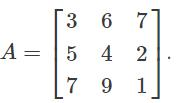Solution:

According to the property of the Eigenvalues, the sum of the Eigenvalues of a matrix is its trace that is the sum of the elements of the principal diagonal.
Therefore, the sum of the Eigenvalues = 3 + 4 + 1 = 8.

QUESTION: 2

### Find the Eigenvalues of matrix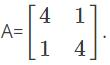Solution:

According to the property of the Eigenvalue, the eigenvalues are determined as follows:
4 + 4 = 8
1+ 1 = 2
The sum of the Eigenvalues is equal to the sum of the principal diagonal elements of the matrix.

QUESTION: 3

### All the four entries of the 2 × 2 matrix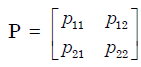are nonzero, and one of its eigen values is zero. Which of the following statements is true?

Solution:

One eigen value is zero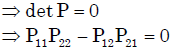QUESTION: 4

The eigen values of the following matrix are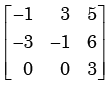Solution:

Let the matrix be A.  We know, Trace (A)=sum of eigen values.

QUESTION: 5

The three characteristic roots of the following matrix A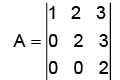Solution:

A is lower triangular matrix. So eigen values are  only the diagonal elements.

QUESTION: 6

The sum of the eigenvalues of the matrix given below is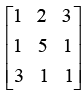Solution:

Sum of eigen values of  A= trace (A)

QUESTION: 7

For which value of x will the matrix given below become singular?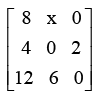Solution:

Let the given matrix be A.  A is singular.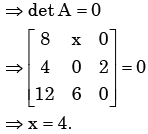QUESTION: 8

Eigen values of a matrix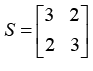are 5 and 1. What are the eigen values of the matrix S2  = SS?

Solution:

We know If λ be the eigen value of A ⇒λ2 is an eigen value of A2 .

QUESTION: 9

The number of linearly independent eigenvectors of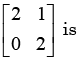Solution:

Number of linear independent vectors is equal to the sum of Geometric Multiplicity of eigen values. Here only eigen value is 2. To find Geometric multiplicity find n-r of (matrix-2I), where n is order and r is rank. Rank of obtained matrix is 1 and n=2 so n-r=1. Therefore the no of linearly independent eigen vectors is 1

QUESTION: 10

The eigenvectors of the matrix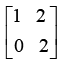are written in the form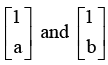. What is a + b?

Solution: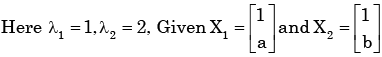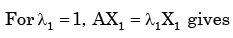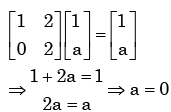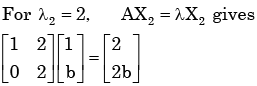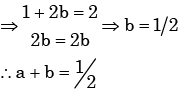QUESTION: 11

One of the Eigenvectors of the matrix A =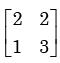is

Solution:

The eigen vectors of A are given by  AX= λ X

So we can check by multiplication.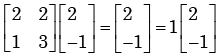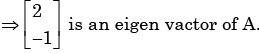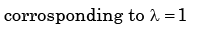QUESTION: 12

The minimum and the maximum eigen values of the matrix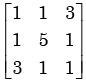are –2 and 6, respectively. What  is the other eigen value?

Solution: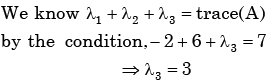QUESTION: 13

The state variable description of a linear autonomous system is, X= AX,

Where X is the two dimensional state vector and A is the system matrix given by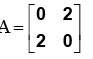The roots of the characteristic equation are

Solution:

Characteristic equation will be :λ2 -4 =0 thus root of characteristic equation will be +2 and - 2.

QUESTION: 14

For the matrix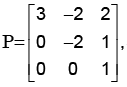s one of the eigen values is equal to -2. Which of the following  is an eigen vector?

Solution: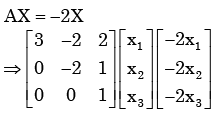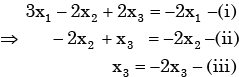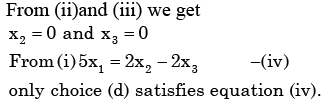QUESTION: 15

x=[x1x2…..xn]T is an n-tuple nonzero vector. The n×n matrix V=xxT

Solution:

As every minor of order 2 is zero.

QUESTION: 16

Cayley - Hamiltion Theorem states that square matrix satisfies its own characteristic equation, Consider a matrix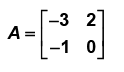A9 equals

Solution: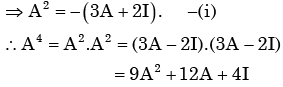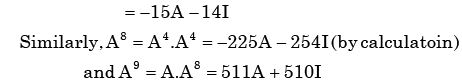QUESTION: 17

If the rank of a (5×6) matrix Q is 4, then which one of the following statements is correct?

Solution:

Rank of a matrix is equal to the No. of linearly independent row or no. of linearly  independent column vector.

QUESTION: 18

The trace and determinate of a 2 ×2 matrix are known to be – 2 and – 35 respectively. Its eigenvalues are

Solution: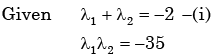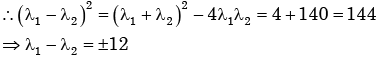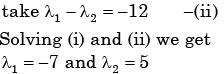QUESTION: 19

Identify which one of the following is an eigenvector of the matrix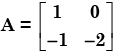Solution:

Eigen Value (λ ) are 1,− 2.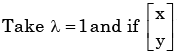be the eigen  of A. Corresponding

To λ then.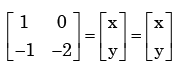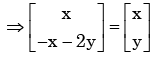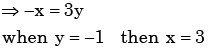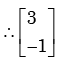be the eigen vector corrosponding to λ = 1

QUESTION: 20

The eigenvalues of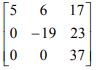Solution:

The eigenvalues of an upper triangular matrix are simply the diagonal entries of the matrix.

Hence 5, -19, and 37 are the eigenvalues of the matrix. Alternately, look atd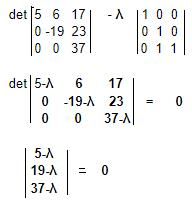λ = 5, -19, 37